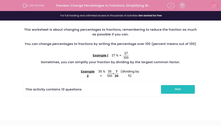# Change Percentages to Fractions, Simplifying Where Possible

In this worksheet, students change percentages to fractions, reducing the fractions as much as possible.Key stage:  KS 2

Curriculum topic:   Maths and Numerical Reasoning

Curriculum subtopic:   Percentages

Difficulty level:#### Worksheet Overview

This worksheet is about changing percentages to fractions, remembering to reduce the fraction as much as possible if you can.

You can change percentages to fractions by writing the percentage over 100 (percent means out of 100)

Example 1  27 % =
 27 100

Sometimes, you can simplify your fraction by dividing by the largest common factor.

Example 2  35 % =
 35 100
=
 7 20
(dividing by 5)

### What is EdPlace?

We're your National Curriculum aligned online education content provider helping each child succeed in English, maths and science from year 1 to GCSE. With an EdPlace account you’ll be able to track and measure progress, helping each child achieve their best. We build confidence and attainment by personalising each child’s learning at a level that suits them.

Get started